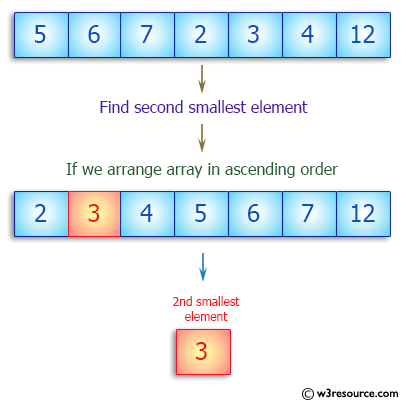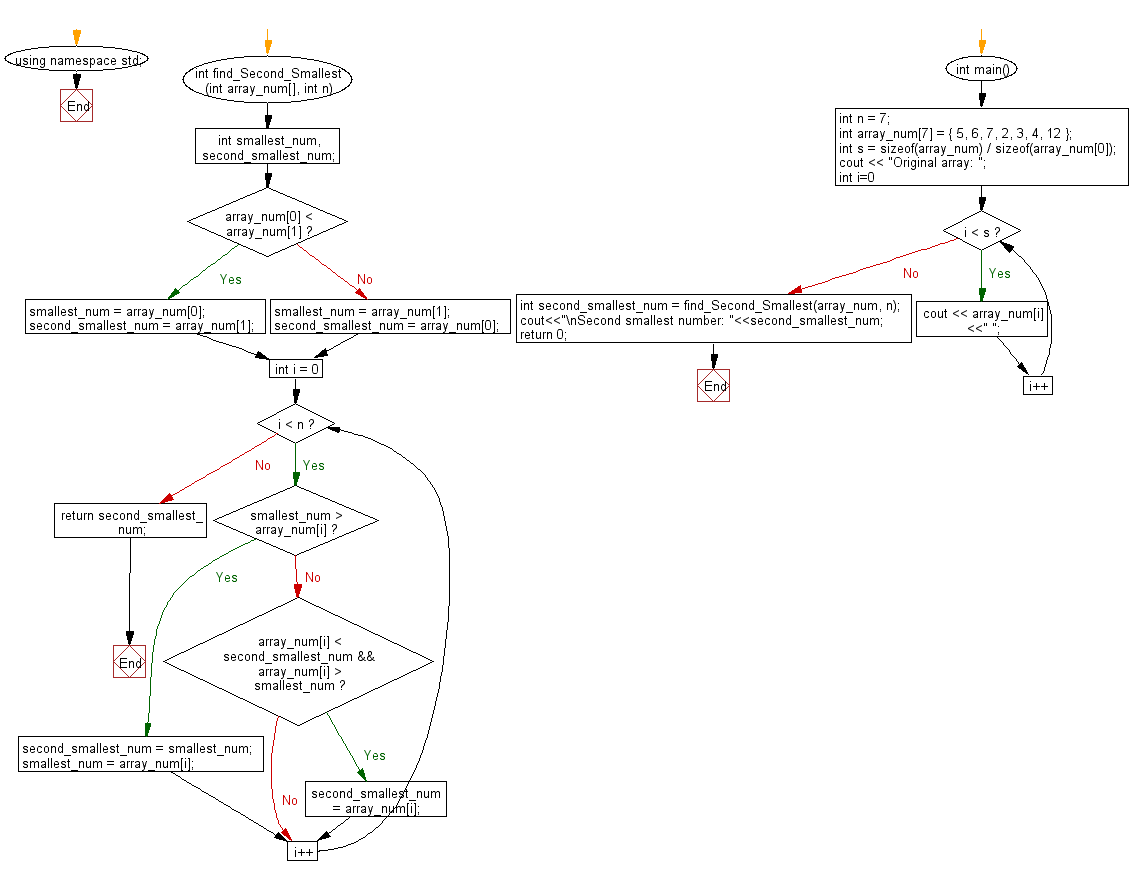﻿ C++ : Find the second smallest elements in an array# C++ Exercises: Find the second smallest elements in a given array of integers

## C++ Array: Exercise-5 with Solution

Write a C++ program to find the second smallest elements in a given array of integers.

Pictorial Presentation:Sample Solution:

C++ Code :

``````#include <iostream>
using namespace std;
int find_Second_Smallest(int array_num[], int n) {
int smallest_num, second_smallest_num;
if (array_num < array_num) {
smallest_num = array_num;
second_smallest_num = array_num;
} else {
smallest_num = array_num;
second_smallest_num = array_num;
}
for (int i = 0; i < n; i++) {
if (smallest_num > array_num[i]) {
second_smallest_num = smallest_num;
smallest_num = array_num[i];
} else if (array_num[i] < second_smallest_num && array_num[i] > smallest_num) {
second_smallest_num = array_num[i];
}
}
return second_smallest_num;
}
int main() {
int n = 7;
int array_num = {
5,
6,
7,
2,
3,
4,
12
};
int s = sizeof(array_num) / sizeof(array_num);
cout << "Original array: ";
for (int i=0; i < s; i++)
cout << array_num[i] <<" ";
int second_smallest_num = find_Second_Smallest(array_num, n);
cout<<"\nSecond smallest number: "<<second_smallest_num;
return 0;
}
``````

Sample Output:

```Original array: 5 6 7 2 3 4 12
Second smallest number: 3
```

Flowchart:C++ Code Editor:

What is the difficulty level of this exercise?

Test your Programming skills with w3resource's quiz.

﻿